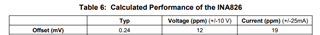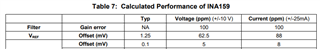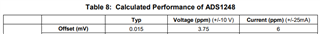If you have a related question, please click the "Ask a related question" button in the top right corner. The newly created question will be automatically linked to this question.

# TIPD164: Offset error for Total Unadjusted Error

Part Number: TIPD164

Dear TI's experts,

i cannot get the results of  the Offset (mV) shown Table 6-8 (https://www.ti.com/lit/ug/tidu491/tidu491.pdf?ts=1630943856512&ref_url=https%253A%252F%252Fwww.ti.com%252Fsolution%252Fanalog-input-module)How to obtain, for example from table 6, the value 12 Voltage (ppm) (+/-10 V) and 19 Current (ppm) (+/-25mA) from 0.24 Typ. Could you explaine me which equation have been used to calculate these Offset (mV) vaules in table 6, 7 and 8 for Voltage and Current as ppm.

Regards,

Davut

• I will be more precise

According table 6: Voffset,volt= 0.24/20*1e3 = 12ppm and Voffset,current = 0.24/(50e-3*249)*1e3 = 19.2771ppm

However, the values in table 7 could not established (for current) in these way: Voffset,volt= 1.25/20*1e3 = 62.5ppm and Voffset,current = 1.25/(50e-3*249)*1e3 = 100.4016ppm this value is 88ppm in the table.

According table 8: Voffset,volt= 0.015/(20*0.2)*1e3 = 3.75ppm and Voffset,current = 0.015/(50e-3*249*0.2)*1e3 = 6.0241ppm. What I don't understand is this last calculation. Why is the voltage ref value +2.5V applied to input AIN0 not been used (Voffset,volt=Voffset,current = 0.015/2.5*1e3 = 6ppm)?

Regards,

Davut

• Hi Davut,